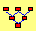Example 3:  European and American Options

Exercise:  In a three-period binomial world, explain the difference between the values of European and American options.

To conduct this exercise, select the “Binomial Tree” module from the Virtual Classroom:

You will see a three-period binomial tree displayed with the following Action and Display Screen.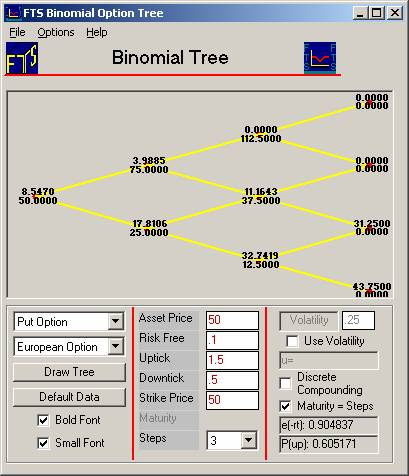The tree displayed is that of the underlying asset, which in this problem we will interpret as a stock.   You can see that the stock price is initially 50, and then moves to either 75 or down to 25, and so on.  The default values have the strike price also at 50, so the options are “at the money.”

Let us look at the trees for European and American put options using the default parameters.  The above screen has the default provided for the European Option (see drop down above).  Below we depict the equivalent screen for the American put option by changing the drop down to American.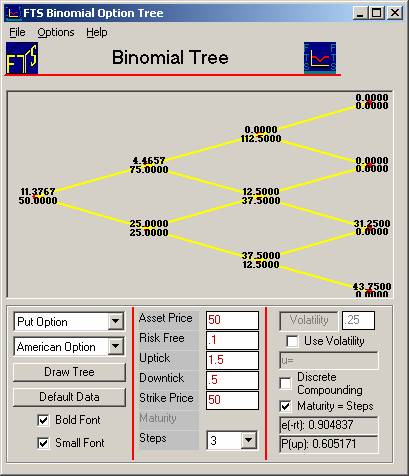You can see that the European put is valued at 8.547 while the American put is worth 11.377.  Comparing the trees, you can see that they differ along the bottom-most path.  After two downticks, the European put is worth 32.742 while the American put is worth 37.50.  At this node, the stock price is 12.5, so the intrinsic value of the put is 37.50.  The numbers in the tree show you that if you do not exercise the put, it is worth 32.742 while if you exercise it, it is worth 37.50. Consequently, it is “rational” to exercise the put before maturity.

Let us verify this by calculating the value of the put if you did not exercise.  In the next period, the put would be worth either 43.75 (if there was a further downtick) or 31.25 (if there was a subsequent uptick).  The risk neutral probability is given by the formula: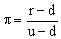Here, r is 1 plus the risk free interest rate, u is the uptick parameter and d is the downtick parameter.  For our values, r =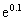, u = 2, and d = .5.  This implies that p = 0.605171 (as shown in the action window).  Using the principle of risk-neutral valuation, we find that the value of the put option at the node is: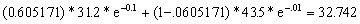Therefore, the difference between the European and American option values is caused by the fact that after two downticks, you would exercise the American style option.

©2003 OS Financial Trading System